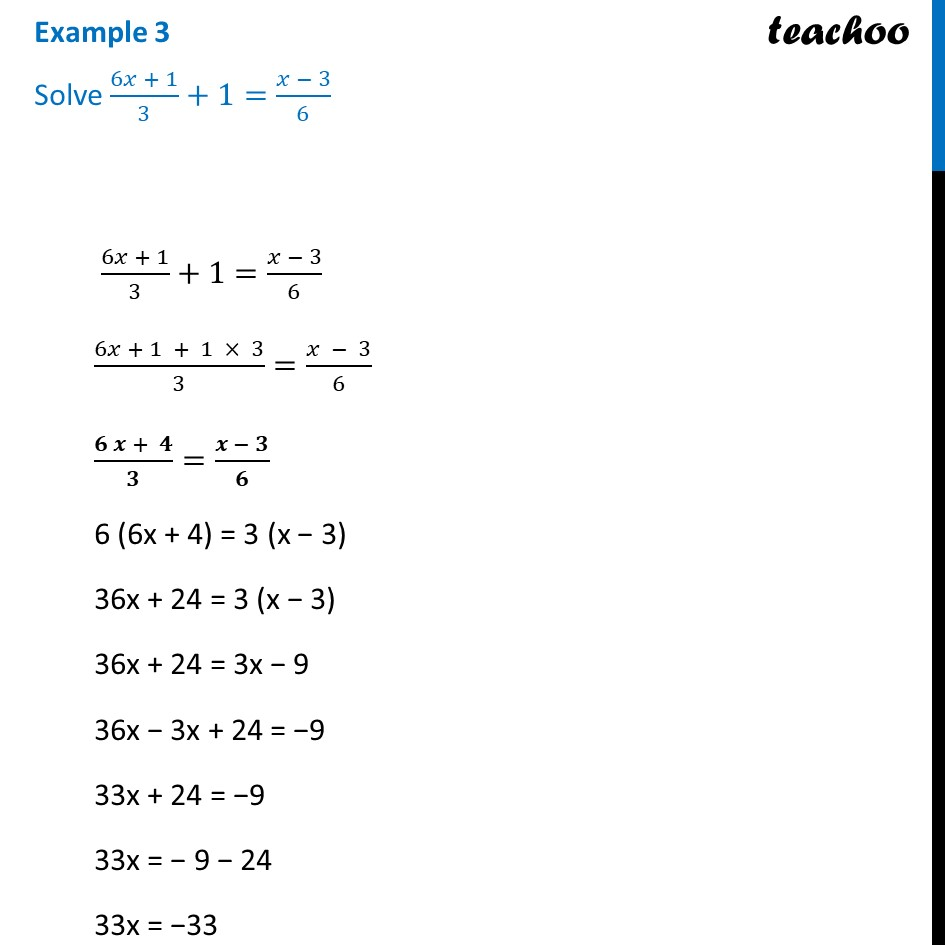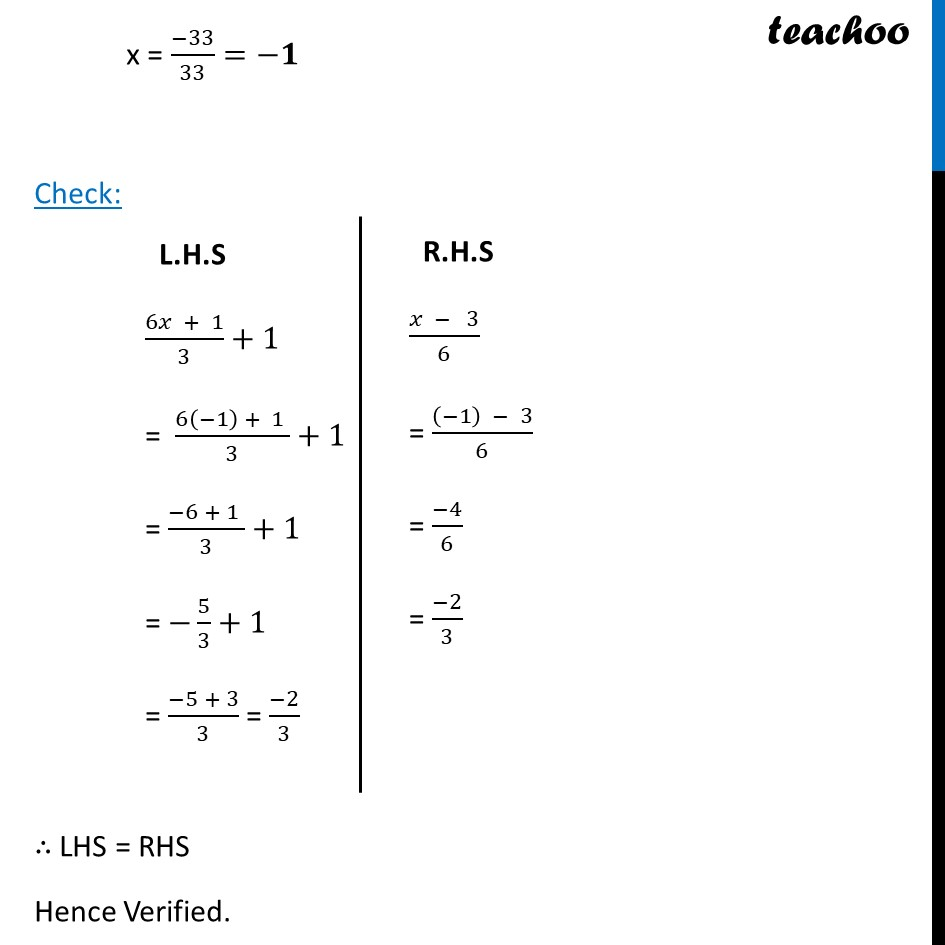Examples

Chapter 2 Class 8 Linear Equations in One Variable
Serial order wiseLearn in your speed, with individual attention - Teachoo Maths 1-on-1 Class

### Transcript

Example 3 Solve (6𝑥 + 1)/3+1=(𝑥 − 3)/6 (6𝑥 + 1)/3+1=(𝑥 − 3)/6 (6𝑥 + 1 + 1 × 3)/3=(𝑥 − 3)/6 (𝟔 𝒙 + 𝟒)/𝟑=(𝒙 − 𝟑)/𝟔 6 (6x + 4) = 3 (x − 3) 36x + 24 = 3 (x − 3) 36x + 24 = 3x − 9 36x − 3x + 24 = −9 33x + 24 = −9 33x = − 9 − 24 33x = −33 L.H.S (6𝑥 + 1)/3+1 = (6(−1) + 1 )/3+1 = (−6 + 1 )/3+1 = −5/3+1 = (−5 + 3)/3 = (−2)/3 x = (−33)/33=−𝟏 Check: ∴ LHS = RHS Hence Verified. R.H.S (𝑥 − 3)/6 = ((−1) − 3)/6 = (−4)/6 = (−2)/3